# cyclebasis

## 语法

``cycles = cyclebasis(G)``
``[cycles,edgecycles] = cyclebasis(G)``

## 说明

``cycles = cyclebasis(G)` 计算无向图的基础循环基。输出 `cycles` 是一个元胞数组，指示哪些节点属于哪个基础循环。 `

``[cycles,edgecycles] = cyclebasis(G)` 还返回每个循环中的边。输出 `edgecycles` 是一个元胞数组，其中 `edgecycles{k}` 给出 `cycles{k}` 中节点之间的边。`

## 示例

```s = [1 1 2 2 3 4 4 5 5 6 7 8]; t = [2 4 3 5 6 5 7 6 8 9 8 9]; G = graph(s,t); plot(G)````cycles = cyclebasis(G)`
```cycles=4×1 cell array {[1 2 5 4]} {[2 3 6 5]} {[4 5 8 7]} {[5 6 9 8]} ```

```s = [1 1 1 2 2 3 3 4 4 4 5 6]; t = [2 3 4 4 5 4 6 5 6 7 7 7]; G = graph(s,t); plot(G);```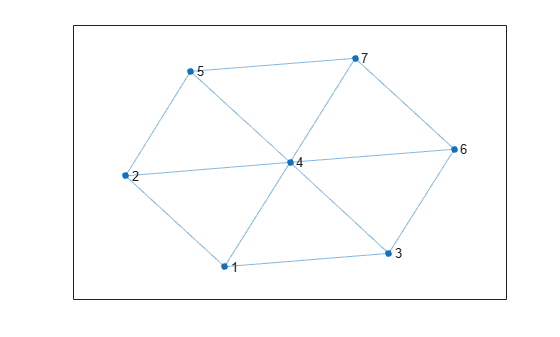`[cycles,edgecycles] = cyclebasis(G)`
```cycles=6×1 cell array {[1 2 4]} {[1 3 4]} {[2 4 5]} {[3 4 6]} {[4 5 7]} {[4 6 7]} ```
```edgecycles=6×1 cell array {[ 1 4 3]} {[ 2 6 3]} {[ 4 8 5]} {[ 6 9 7]} {[8 11 10]} {[9 12 10]} ```

```tiledlayout flow for k = 1:length(cycles) nexttile highlight(plot(G),cycles{k},'Edges',edgecycles{k},'EdgeColor','r','NodeColor','r') title("Cycle " + k) end```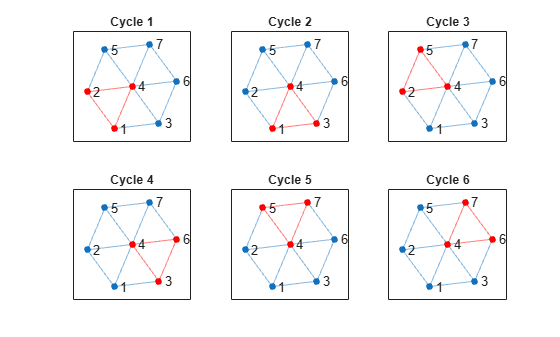```figure new_cycle_edges = setxor(edgecycles{1},edgecycles{2}); highlight(plot(G),'Edges',new_cycle_edges,'EdgeColor','r','NodeColor','r')``````n = 5; A = delsq(numgrid('S',n)); G = graph(A,'omitselfloops'); plot(G)```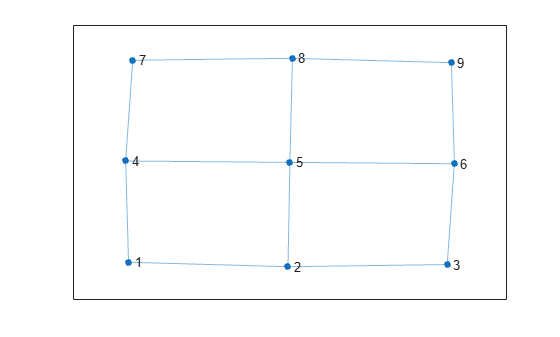```[cycles,edgecycles] = allcycles(G); tiledlayout flow for k = 1:length(cycles) nexttile highlight(plot(G),cycles{k},'Edges',edgecycles{k},'EdgeColor','r','NodeColor','r') title("Cycle " + k) end```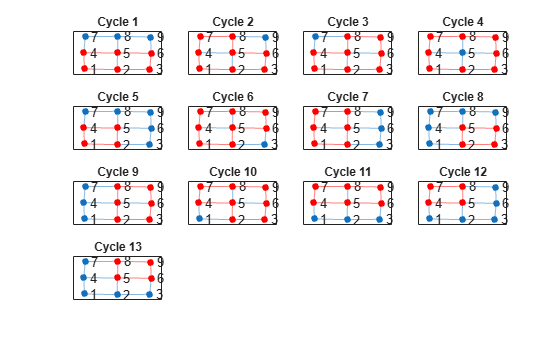```[cycles,edgecycles] = cyclebasis(G); tiledlayout flow for k = 1:length(cycles) nexttile highlight(plot(G),cycles{k},'Edges',edgecycles{k},'EdgeColor','r','NodeColor','r') title("Cycle " + k) end```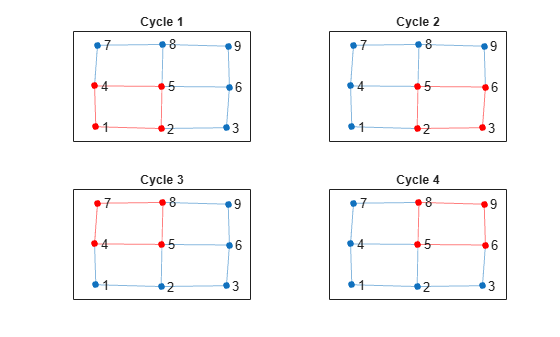```n = 6; A = delsq(numgrid('S',n)); G = graph(A,'omitselfloops'); figure plot(G)```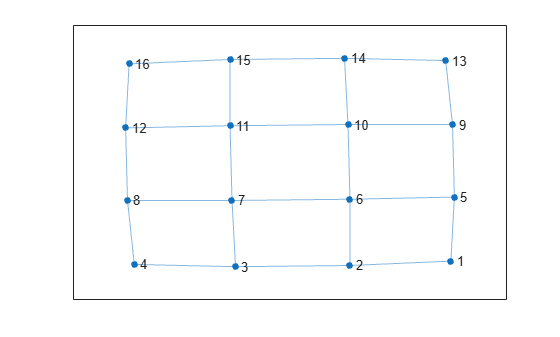`allcycles(G)`
```ans=213×1 cell array {[ 1 2 3 4 8 7 6 5]} {[ 1 2 3 4 8 7 6 10 9 5]} {[1 2 3 4 8 7 6 10 11 12 16 15 14 13 9 5]} {[ 1 2 3 4 8 7 6 10 11 15 14 13 9 5]} {[ 1 2 3 4 8 7 6 10 14 13 9 5]} {[ 1 2 3 4 8 7 11 10 6 5]} {[ 1 2 3 4 8 7 11 10 9 5]} {[ 1 2 3 4 8 7 11 10 14 13 9 5]} {[ 1 2 3 4 8 7 11 12 16 15 14 10 6 5]} {[ 1 2 3 4 8 7 11 12 16 15 14 10 9 5]} {[ 1 2 3 4 8 7 11 12 16 15 14 13 9 5]} {[1 2 3 4 8 7 11 12 16 15 14 13 9 10 6 5]} {[ 1 2 3 4 8 7 11 15 14 10 6 5]} {[ 1 2 3 4 8 7 11 15 14 10 9 5]} {[ 1 2 3 4 8 7 11 15 14 13 9 5]} {[ 1 2 3 4 8 7 11 15 14 13 9 10 6 5]} ⋮ ```

```[cycles,edgecycles] = cyclebasis(G); figure tiledlayout flow for k = 1:length(cycles) nexttile highlight(plot(G),cycles{k},'Edges',edgecycles{k},'EdgeColor','r','NodeColor','r') title("Cycle " + k) end```## 输出参数

`G` 中的每个循环均为在 `cycles` 中返回的基础循环的组合。如果一条边是 `G` 中某循环的一部分，则它也是 `cycles` 中至少一个基础循环的一部分。

`cycles` 中元胞的数据类型取决于输入图是否包含节点名称：

• 如果图 `G` 没有节点名称，则每个元胞 `cycles{k}` 均为节点索引的数值向量。

• 如果图 `G` 有节点名称，则每个元胞 `cycles{k}` 是字符向量节点名称的元胞数组。

## 详细信息

### 基础循环基

`cyclebasis` 中使用的最小生成树通常不同于 `minspantree` 返回的生成树。它的选择条件是使循环尽可能短。但是，`cyclebasis` 无法保证返回最短的基础循环。